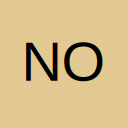# Count Replacements in VBA

• Hi All,

I have the following code.

Code
1. Cells.Replace What:=ActiveSheet.Name & "!", Replacement:="", LookAt:=xlPart, _
2. SearchOrder:=xlByRows, MatchCase:=False, SearchFormat:=False, _
3. ReplaceFormat:=False

If I do a standard Find & Replace then excel will display a msgbox displaying the number of replacements made. I'm struggling to figure out the VBA needed to count the number of replacements so that I can put a msgbox together to alert the user of the number of replacements made.

Appreciate any help given on this topic.

Kindest regards,
Jon

• Re: Count Replacements in VBA

This code will find A and replace with B & give a count

• Re: Count Replacements in VBA

Thanks very much Roy. Not sure what i is doing though, sorry, I'm still trying to learn this.

I my spreadsheet this is changing 1 cell yet the count is 121? Could you help me understand this please?

Many thanks,
Jon

• Re: Count Replacements in VBA

Thanks again Roy.

I'm trying to build a macro that will remove the active worksheet name from all exisitng formula in the active worksheet. This is because though I prefer to use short names for my worksheets, someone senior to me has insisted that we use more "meaningful" names and these are often very long. Therefore, if we reference several cells from multiple worksheets in a formula and then reference the active worksheet in that same forumla, the characters occassionally exceed the number allowed for in Excel because it inserts the active worksheet name unneccessarily. I'm attempting to build a turnkey operation to shorten this.

Is there any other means of overcoming this problem that you can think of?

Gratefully,
Jon

• Re: Count Replacements in VBA

Ok, it's not crucial so I'll make do without. Many thanks for your help.

• Re: Count Replacements in VBA

This code will find A and replace with B & give a count

This is incorrect. That example actually only counts the number of cells in the used range. It does not count what cells had something found and replaced.

You need something like this: https://stackoverflow.com/a/33627977/1898524

• You could try

Code
1. Sub Maybe()
2. Dim c As Range, i As Long
3. i = 0
4. For Each c In ActiveSheet.UsedRange.SpecialCells(xlCellTypeFormulas)
5. If InStr(c.Formula, ActiveSheet.Name) > 0 Then
6. c.Formula = Replace(c.Formula, ActiveSheet.Name & "!", ""): i = i + 1
7. End If
8. Next c
9. MsgBox i
10. End Sub